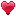• ようこそゲストさん！

### bbbcさんの日記

（SNS全体・外部に公開（Web全体に公開））

2017年
11月22日
14:54TED-Ed0808　How to prove the Pythagorean theorem?　　　　（ ピタゴラスの定理の証明方法 ）

代表頁に戻る
埋め込みYouTubeのブラウザは Edge Chrome I E は対応していない。

05分　135wpm

.　　　　 動画を見るとき、 でフルスクリーンに拡大すると見やすい。

What do Euclid, twelve-year-old Einstein, and American President James Garfield have in common? They all came up with elegant proofs for the famous Pythagorean theorem, the rule that says for a right triangle（直角三角形）, the square of one side plus the square of the other side is equal to the square of the hypotenuse（斜辺 [hɑip:tənu:s] ）.
発音注意　 Pythagorean ：[piθægəríən]. ピタゴラスの
Pythagoras　：[piθǽgərəs] 　ピタゴラス

In other words, a²+b²=c² （c squared）. This statement is one of the most fundamental rules of geometry, and the basis for practical applications, like constructing stable buildings and triangulating（三角測量すること） GPS coordinates（GPS座標）.

The theorem is named for Pythagoras, a Greek philosopher and mathematician in the 6th century B.C., but it was known more than a thousand years earlier. A Babylonian tablet from around 1800 B.C. lists 15 sets of numbers that satisfy the theorem.

Some historians speculate that Ancient Egyptian surveyors（測量士） used one such set of numbers, 3, 4, 5, to make square corners. The theory is that surveyors could stretch a knotted rope with twelve equal segments to form a triangle with sides of length 3, 4 and 5. According to the converse（逆） of the Pythagorean theorem, that has to make a right triangle, and, therefore, a square corner.

And the earliest known Indian mathematical texts written between 800 and 600 B.C. state that a rope stretched across the diagonal（対角線） of a square produces a square twice as large as the original one.

That relationship can be derived from the Pythagorean theorem. But how do we know that the theorem is true for every right triangle on a flat surface, not just the ones these mathematicians and surveyors knew about? Because we can prove it. Proofs use existing mathematical rules and logic to demonstrate that a theorem must hold true all the time.

One classic proof often attributed to Pythagoras himself uses a strategy called proof by rearrangement. Take four identical right triangles with side lengths a and b and hypotenuse length c. Arrange them so that their hypotenuses form a tilted square.
The area of that square is c². Now rearrange the triangles into two rectangles（矩形）, leaving smaller squares on either side. The areas of those squares are a² and b². Here's the key. The total area of the figure didn't change, and the areas of the triangles didn't change. So the empty space in one, c² must be equal to the empty space in the other, a² + b².

Another proof comes from a fellow Greek mathematician Euclid and was also stumbled upon almost 2,000 years later by twelve-year-old Einstein. This proof divides one right triangle into two others and uses the principle that if the corresponding angles of two triangles are the same, the ratio of their sides is the same, too.　So for these three similar（相似の） triangles, you can write these expressions for their sides. Next, rearrange the terms. And finally, add the two equations together and simplify to get ab²+ac²=bc², or a²+b²=c².

Here's one that uses tessellation（平面充填）, a repeating geometric pattern for a more visual proof. Can you see how it works? Pause the video if you'd like some time to think about it. Here's the answer. The dark gray square is a² and the light gray one is b². The one outlined in blue is c². Each blue outlined square contains the pieces of exactly one dark and one light gray square, proving the Pythagorean theorem again.
この平面充填はインド発祥の証明法。 三角形の合同パスルのようにややこしい。

And if you'd really like to convince yourself, you could build a turntable with three square boxes of equal depth connected to each other around a right triangle. If you fill the largest square with water and spin the turntable, the water from the large square will perfectly fill the two smaller ones. The Pythagorean theorem has more than 350 proofs, and counting, ranging from brilliant to obscure（あいまいな）. Can you add your own to the mix?
-
• 総アクセス数(1,525)
•拍手(1)
•お気に入り(1)

### コメント

1番～3番を表示

2017年
11月25日
05:351:

>> was also stumbled upon almost 2,000 years later by twelve-year-old Einstein.
アインシュタインは、子供の頃、劣等生、特に、数学は全くできなかったそうです。

この英文は、その通りのことを書いてあると思います。
リーマン幾何学では、地球の赤道を平行に切る線が、北極で交わる（ユークリッド幾何学では、平行線は交わらないという説）というもの。
このコメントは蛇足？

2017年
11月25日
08:03>>1 BlauerHimmelさん コメントありがとうございます。

赤道から直角に出る線（経度の線）は何本も描ける平行線だが、
この平行線は 北極と南極で交わる。

ユークリッド幾何学は「平行線は交わらない」 が 代表的性質。
（まっすぐな空間の幾何学）
それ以外が 非ユークリッド幾何学 と いうことになる。
（曲がった空間の幾何学）
リーマン幾何学は、非ユークリッド幾何学の一つで多次元の幾何学。
これ以上は数学の分野ですね・・・・。

2017年
11月26日
03:563:

>>1 BlauerHimmelさん

ほかの、いろいろな理論も、証明してもらえないと、正しい理論と言えないので。

was also stumbled upon　　ピタゴラスの定理の証明を、たまたま思いついたけれど、数学的に証明する能力がなかったので、リーマンという数学者に依頼したとか。（もっとも、ピタゴラスの定理のことではなさそうですが）。

それにしても、物理と数学とは、別なんだな　ということと、１２歳で、数学劣等生が、こんなことを思いつくなんて、びっくり。

1番～3番を表示